Function Repository Resource:

# BinarySearchBy

Find the position of a target value within an array sorted after applying a function

Contributed by: Wolfram Staff
 ResourceFunction["BinarySearchBy"][list,val,f] gives the position of the last element ei in list such that f[ei]≤val.

## Details and Options

ResourceFunction["BinarySearchBy"][list,val,f] is equivalent to BinarySearch[f/@list,val].
Elements of f/@list must be numeric.
ResourceFunction["BinarySearchBy"][list,val,f] assumes that f/@list is arranged in ascending order and can give an erroneous result if it is not.

## Examples

### Basic Examples (1)

Find the position of the last pair whose first element is not greater than 5:

 In:=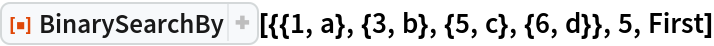Out=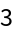### Properties and Relations (1)

BinarySearchBy is essentially equivalent to the resource function BinarySearch after applying f to elements of list:

 In:=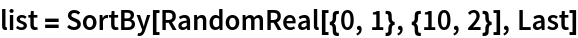Out=In:=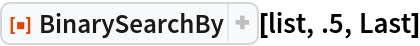Out=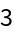In:=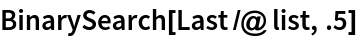Out=### Possible Issues (2)

BinarySearchBy[list,val,f] can give an erroneous result if elements obtained by applying f to list are not arranged in ascending order:

 In:=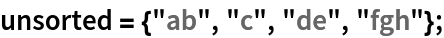In:=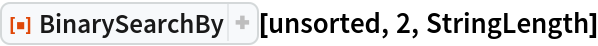Out=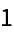Get the result after sorting the list:

 In:=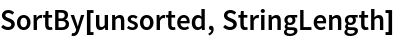Out=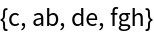In:=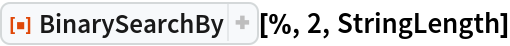Out=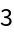## Version History

• 1.0.0 – 09 March 2020